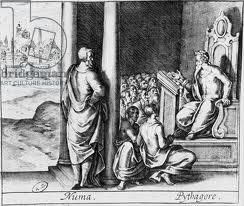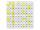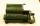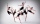# Class

When Pythagoras asked how many students attend the school, said: "Half of the students studying mathematics, 1/4 music, seventh silent and there are three girls at school." How many students had Pythagoras at school?

Correct result:

n =  28

#### Solution:

$n=2\cdot 2\cdot 7=4\cdot 7=28$We would be pleased if you find an error in the word problem, spelling mistakes, or inaccuracies and send it to us. Thank you!## Next similar math problems:

• Roman numeralsWrite numbers written in Roman numerals as decimal.Added together and write as decimal number: LXVII + MLXIV
• DivisionWhich number in division 16 give 12 and the rest 3?
• Write decimalsWrite in the decimal system the short and advanced form of these numbers: a) four thousand seventy-nine b) five hundred and one thousand six hundred and ten c) nine million twenty-six
• Primes 2Which prime numbers is number 2025 divisible?
• Prime factorsWrite 98 as product of prime factors
• Evaluate 5Evaluate expression x2−7x+12x−4 when x=−1
• LCMCommon multiple of three numbers is 3276. One number is in this number 63 times, second 7 times, third 9 times. What are the numbers?
• IntegersMay be the sum of two integers less than their difference?
• The number 3Ski organizers should print the start numbers from 1 to 45. How many times will they use the number 3 when printing?
• NumberDetermine unknown number if you know that difference between five times and triple of number is 42.
• LCM of two numberFind the smallest multiple of 63 and 147
• Divisibility 2How many divisors has integer number 13?
• DigitsHow many odd four-digit numbers can we create from digits: 0, 3,5,6,7?
• Dance ensembleThe dance ensemble took the stage in pairs. During dancing, the dancers gradually formed groups of four, six and nine. How many dancers have an ensemble?
• Sum of the digitsHow many are two-digit natural numbers that have the sum of the digits 9?
• Two-digit numberI am a two-digit number less than 20. When I divided by three, then you get the rest 1 and when you divide me by four you get also rest 1. What number am I?